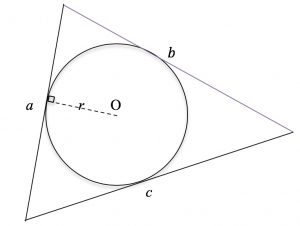A circle of radius r and centre O, is inscribed in a triangle with edges of lengths a, b and c.

Find the area and circumference of the circle.

Find the area and the perimeter of the triangle.

What do you notice about the ratios of the two areas and the ratios of the two perimeters?

What do you notice about the ratio of the perimeter to the area for each shape?

Tagged with: